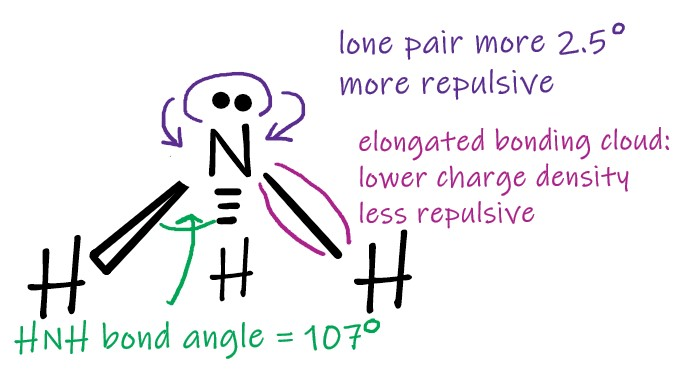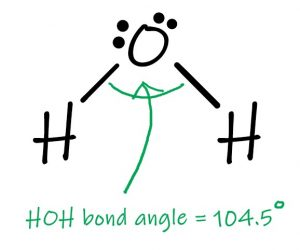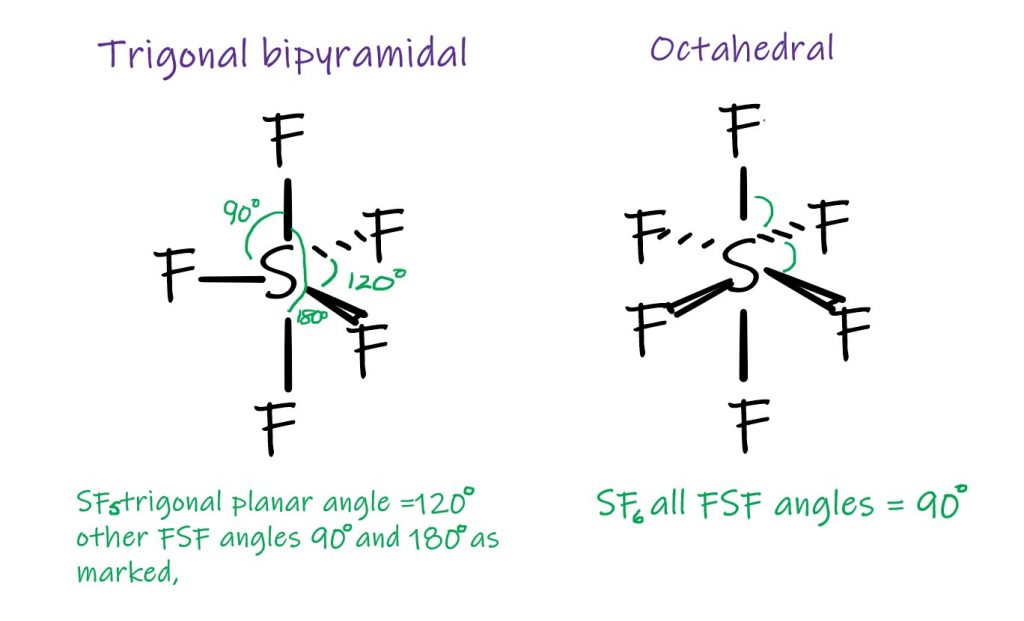Select Page

VSEPR theory (Valence Shell Electron Pair Repusion) is what we use to explain the shapes of molecules in Chemistry.  I have said before that Size Matters in Chemistry, but shape also matters. We can talk about the VSEPR as a starter, all the way up to enzymes, and everything in between when we are discussing shapes of molecules. We write and draw molecules in two dimensions, but it is important to remember that molecules are not two dimensional. And when we do remember that, life can become very interesting indeed. I have to acknowledge that I love Geometry. As a child, maths was my thing, until I formally discovered Chemistry at the age of thirteen. I also like Algebra, I have to confess, but here, it is about geometry, symmetry and pattern.

### VSEPR Theory and the Shapes of Molecules

Shapes of covalent molecules arise because electrons repel each other, on account of their “like” charge. If you have electrons in close proximity to each other they will try to get as far away as they possibly can. In VSEPR theory, we are talking about pairs of electrons in covalent bonds, and also non bonding electrons. We consider the arrangement around the central atom, and start from period 2 elements as being the “ideal” situation. By that, I mean the “octet rule”, where the atom achieves, by sharing, a full outer shell containing eight electrons. Methane is our starting point.

For molecules with three equivalent bonds, the furthest apart the atoms can get from each other is in a trigonal planar arranegment, where the bond and around the central atom is 120 degrees. It is bascially an equilaterial triangle and the molecule is flat.. An example of a molecule with this shape is boron trifluoride.

Where there are two equivalent bonds, the molecule will be linear around the central atom. Carbon dioxide is one such case, and although we are speaking about double bonds rather than single bonds, they are equivalent and will repel each other with equal force.

#### Deviations from the Tetrahedral Arrangement

If the ideal situation is the octet rule, as referred to earlier, we can assume that the tetrahedral is the “ideal” shape around the central atom. For other situations where there are eight electrons around the central atom the non bonding pairs of electrons are important. For example, consider ammonia. Nitrogen has five electrons in its outer shell. It only uses three of them to form bonds with hydrogen. The electron cloud containing the lone pair is short and fat compared to the elongated bonding clouds. The concentration of charge in this shape makes it more repulsive than the bonding pairs.• Pinterest

Ammonia• Pinterest

Water

Water is another example where we see the stronger repulsion of the lone pair of electrons. Here, the HOH bond angle is 104.5 degrees, a devaition of 2 x 2.5 from the tetrahedral bond angle.

### Splitting the Octet

In the third period of the Periodic Table, the central atoms can deviate from the octet rule for the outer shell electrons and as a result can lead to further molecular shapes, still based on VSEPR theory. SF5, where there are five equivalent bonds leads to the trigonal bipyramidal shape. It is a bit like taking the BF3 molecule and putting a F above and below it to make the points two pyramids: one above and one below the molecule. SF6, with its six equivalent bonds give the octahedral shape. It is also like two pyramids joined base to base, but the base is a square, rather than a triangle.• Pinterest

Shapes for 5 and 6 equivalent bonds

In conclusion, for Shape and the VSEPR theory, it is not enough simply to count the covalent bonds around the central atom. We have to consider the total number of electron pairs and whether or not they are involved in bonding. As the name of the theory suggests, it is all about repulsion of electron pairs. The electron clouds are trying to get as far away from each other as possible. Not all electron clouds are equal in terms of the force they can exert. Lone pairs are more repulsive than double bonds, which are in turn more repulsive than single bonds. Here, I have just presented the idealised situations. In reality, the measured bond angles will vary slightly depending on the molecule, but will approximate to the shapes described.